# 使用Matrix做一个图片过渡动画吧！

## 前言## 一、Matrix是什么？

`Matrix` 是一个 3*3 的矩阵，如果你已经忘了矩阵是什么？可以在 【这里】 回顾一下。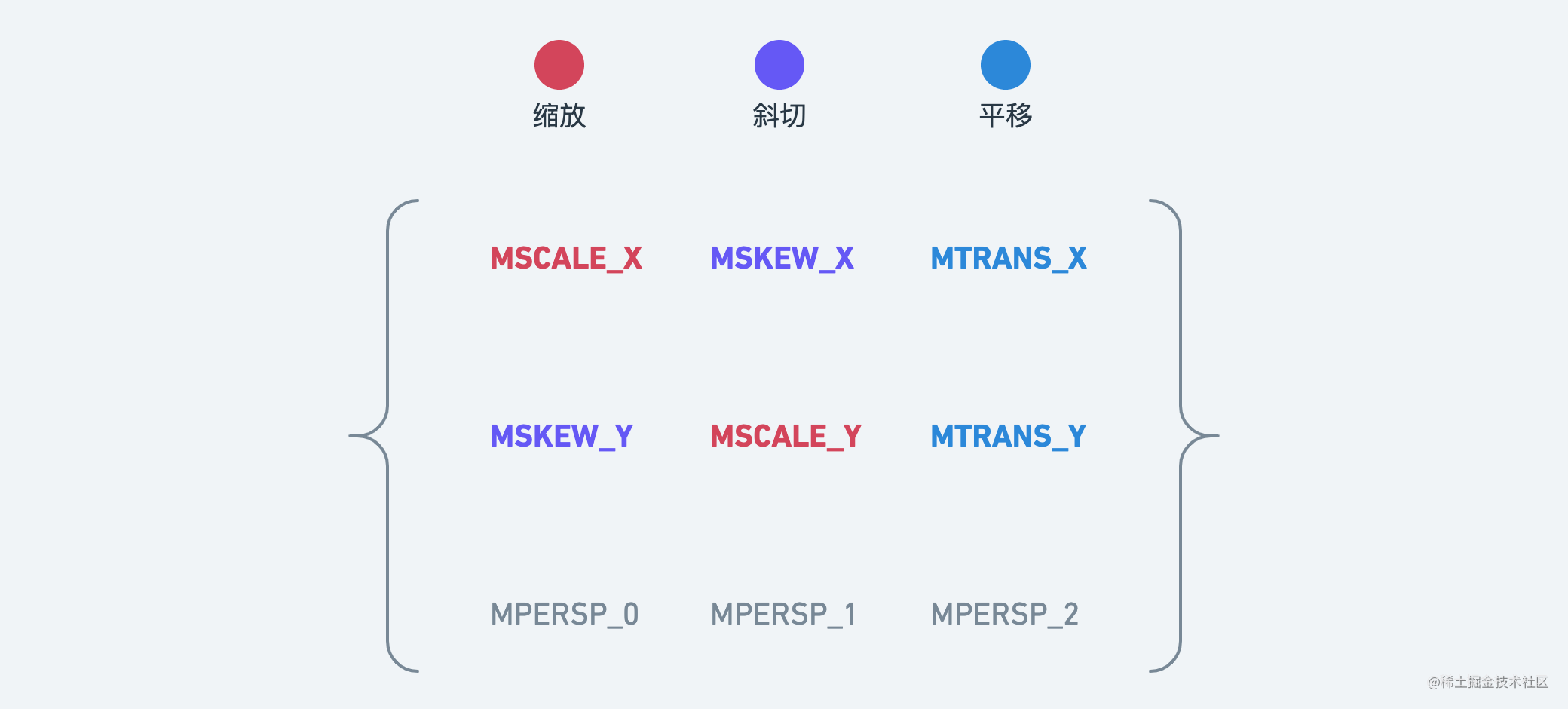### 1.缩放(Scale)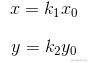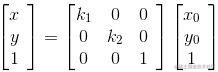(x, y, 1) - 点
(x, y, 0) - 向量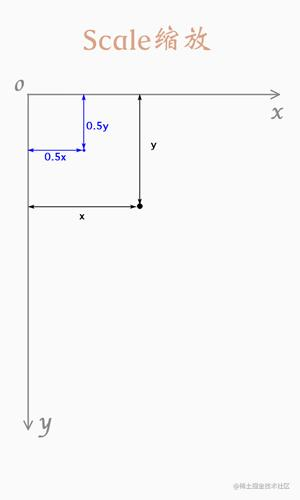### 2.错切(Skew)

#### 水平错切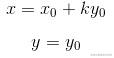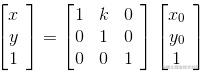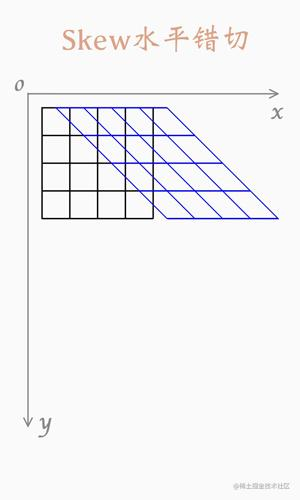#### 垂直错切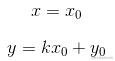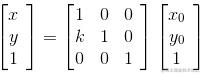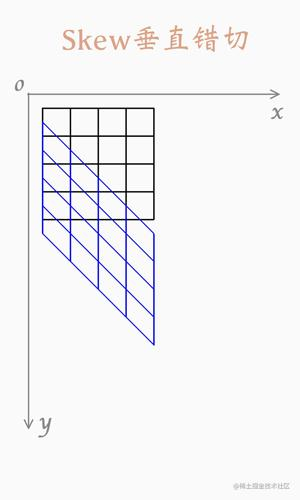#### 复合错切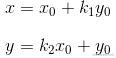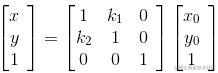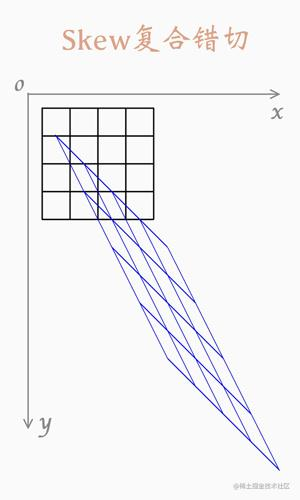### 3.旋转(Rotate)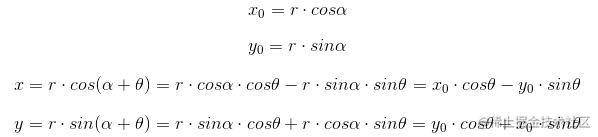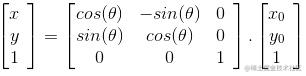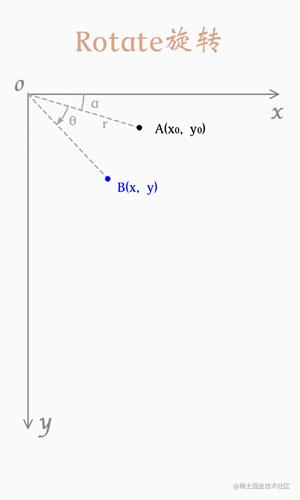### 4.平移(Translate)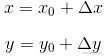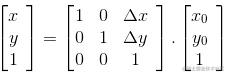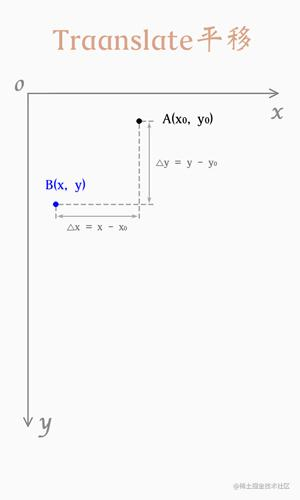## 二、ScaleType和Matrix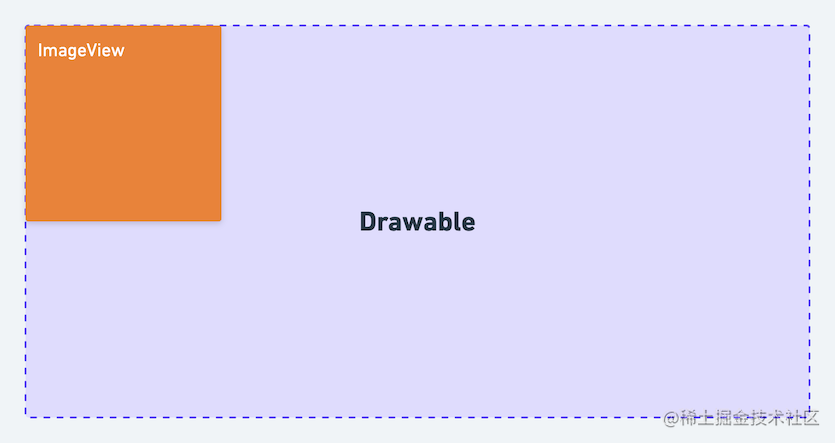### 1. CENTER_CROP

`CENTER_CROP` 是我们平时用的最多的一种类型。规则：图片宽高等比缩放，直到宽高都大于或者等于控件的宽高，图片多出来的部分会被裁切

``````控件长 / 图片长 = o.25

``````[0.5  0    0]
[0    0.5  0]
[0    0    1]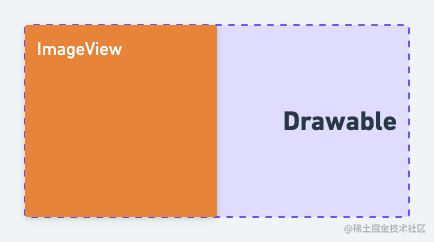`translationX = (imageViewWidth - drawableWidth * scale) / 2`，需要平移矩阵左乘缩放矩阵，更新后的 Matrix 为：

``````[1  0  -150]     [0.5  0  0]    [0.5  0  -150]
[0  1     0]  *  [0  0.5  0] =  [0  0.5     0]
[0  0     1]     [0  0    1]    [0  0       1]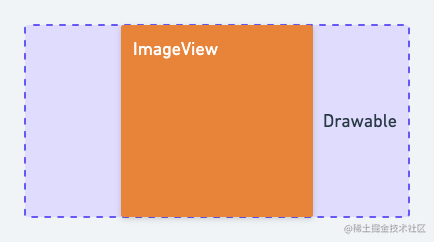### 2. Center

`Center` 规则：图片不缩放，只平移，最终将图片中心区域展示在控件上。

``````translationX = (imageViewWidth - drawableWidth) / 2;
translationY = (imageViewHeight - drawableHeight) / 2;

`Matrix` 为：

``````[1  0  -450]
[0  1  -150]
[0  0     1]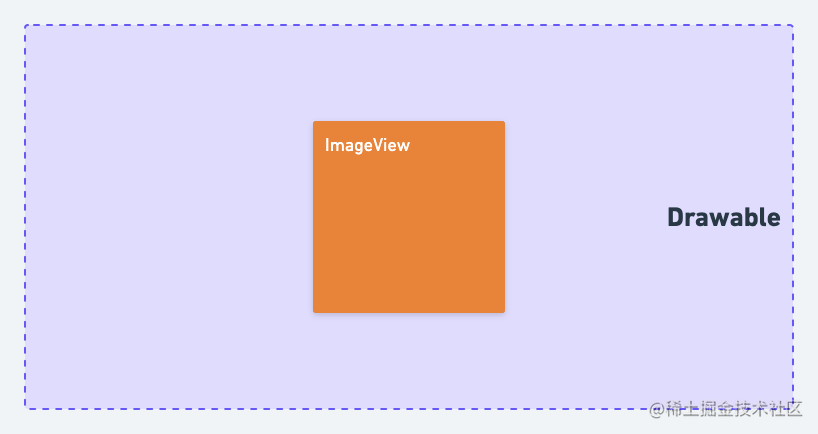### CENTER_INSIDE

`CENTER_INSIDE` 规则：等比缩小图片，使得控件可以展示完整的图片并居中展示，当图片小于控件的宽高时不放大。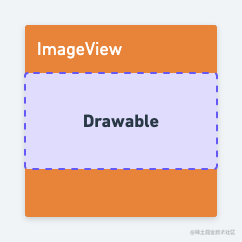### FIT_XY

`FIT_XY` 规则：分别缩放图片宽高，缩放后的宽高会等于控件的宽高，图片可能会变形。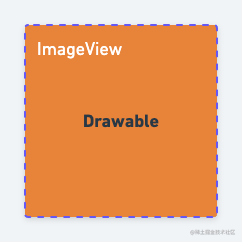### FIT_START

`FIT_START` 规则：等比缩放图片宽高，控件可以完整展示图片，平移至头部区域。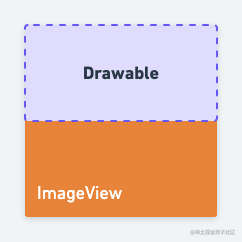### FIT_CENTER

`FIT_CENTER` 规则：等比缩放图片宽高，控件可以完整展示图片，平移至控件中心区域，当图片宽高小于控件宽高的时会对图片放大。### FIT_END

`FIT_END` 规则：等比缩放图片宽高，控件可以完整展示图片，平移到控件底部和尾部。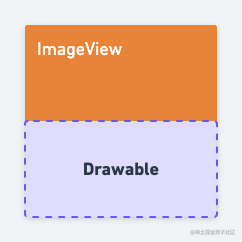### Matrix

`Matrix` 规则：自定义 `Matrix`

## 三、做一个Matrix动画

``````val evaluator = MatrixEvaluator()
val matrix = evaluator.evaluate(it.animatedFraction, firstMatrix, secondMatrix)
iv.imageMatrix = matrix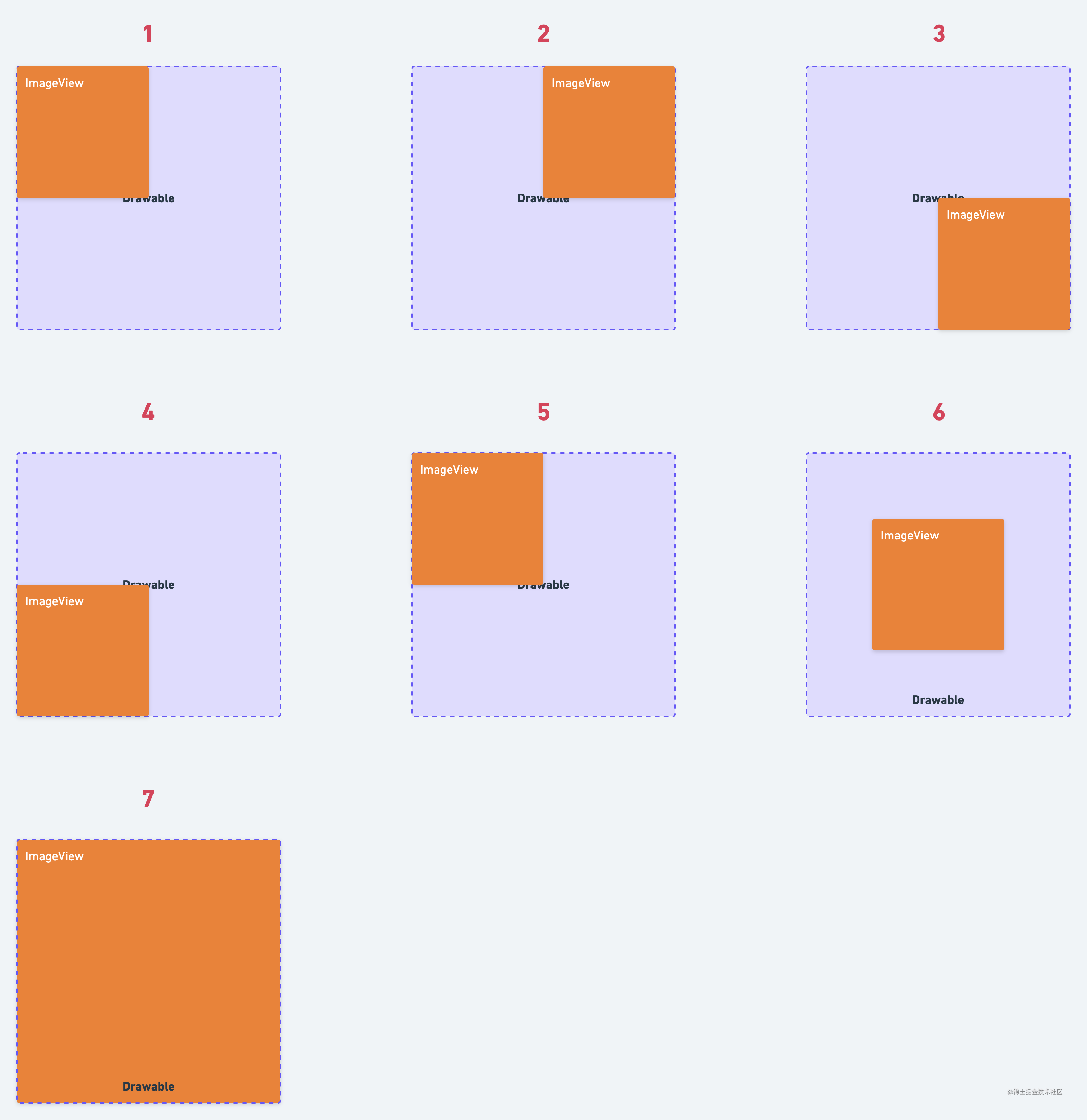1. 第一张：当前 `Matrix` 不变。
2. 第二张：当前 `Matrix` 向右平移一半宽。
3. 第三张：当前 `Matrix` 向右平移一半宽，向下平移一半高。
4. 第四张：当前 `Matrix` 向下平移一半高。
5. 第五张：当前 `Matrix` 同第一张。
6. 第六张：当前 `Matrix` 向右平移1/4宽，向下平移1/4高。
7. 第七张：当前 `Matrix` 不变。

``````fun doSomeThingAnimation() {
ivContent?.let { iv ->
val imgWidth = iv.width
val imgHeight = iv.height
iv.layoutParams.width = imgWidth / 2
iv.layoutParams.height = imgHeight / 2
val tX = imgWidth.toFloat() / 2
val ty = imgHeight.toFloat() / 2
val initMatrix = iv.imageMatrix

// 获取6个Matrix
val oneMatrix = Matrix(initMatrix)
val twoMatrix = Matrix(initMatrix).apply {
postTranslate(-tX, 0f)
}
val threeMatrix = Matrix(initMatrix).apply {
postTranslate(-tX, -ty)
}
val fourthMatrix = Matrix(initMatrix).apply {
postTranslate(0f, -ty)
}
val fiveMatrix = Matrix(initMatrix).apply {
postTranslate(-tX / 2, - ty / 2)
}
val sixMatrix = Matrix(initMatrix)

iv.scaleType = ScaleType.MATRIX
iv.imageMatrix = oneMatrix

// 构建6段动画
val evaluator = MatrixEvaluator()
val firstAnimator  = ValueAnimator.ofFloat(0f, 1f)
val matrix = evaluator.evaluate(it.animatedFraction, oneMatrix, twoMatrix)
iv.translationX = it.animatedFraction  * imgWidth / 2
iv.imageMatrix = matrix
}
val secondAnimator  = ValueAnimator.ofFloat(0f, 1f)
val fraction = it.animatedFraction
iv.translationY = fraction * imgHeight / 2
val matrix = evaluator.evaluate(fraction, twoMatrix, threeMatrix)
iv.imageMatrix = matrix
}
val threeAnimator  = ValueAnimator.ofFloat(0f, 1f)
val fraction = it.animatedFraction
iv.translationX = ((1 - fraction) * imgWidth / 2)
val matrix = evaluator.evaluate((fraction), threeMatrix, fourthMatrix)
iv.imageMatrix = matrix
}
val fourAnimator  = ValueAnimator.ofFloat(0f, 1f)
val fraction = it.animatedFraction
iv.translationY = ((1 - fraction) * imgHeight / 2)
val matrix = evaluator.evaluate((fraction), fourthMatrix, oneMatrix)
iv.imageMatrix = matrix
}
val fiveAnimator  = ValueAnimator.ofFloat(0f, 1f)
val fraction = it.animatedFraction
iv.translationY = (fraction * imgHeight / 4)
iv.translationX = (fraction * imgWidth / 4)
val matrix = evaluator.evaluate((fraction), oneMatrix, fiveMatrix)
iv.imageMatrix = matrix
}
val sixAnimator  = ValueAnimator.ofFloat(0f, 1f)
val fraction = it.animatedFraction
iv.layoutParams.width = (imgWidth / 2 + imgWidth / 2 * fraction).toInt()
iv.layoutParams.height = (imgHeight / 2 + imgHeight / 2 * fraction).toInt()
iv.translationY = ((1 - fraction) * imgHeight / 4)
iv.translationX = ((1 - fraction) * imgWidth / 4)
val matrix = evaluator.evaluate((fraction), fiveMatrix, sixMatrix)
iv.imageMatrix = matrix
iv.requestLayout()
}
val set = AnimatorSet()
set.playSequentially(firstAnimator, secondAnimator, threeAnimator, fourAnimator, fiveAnimator, sixAnimator)
set.duration = 1000
set.start()
}
}

《Matrix简介》